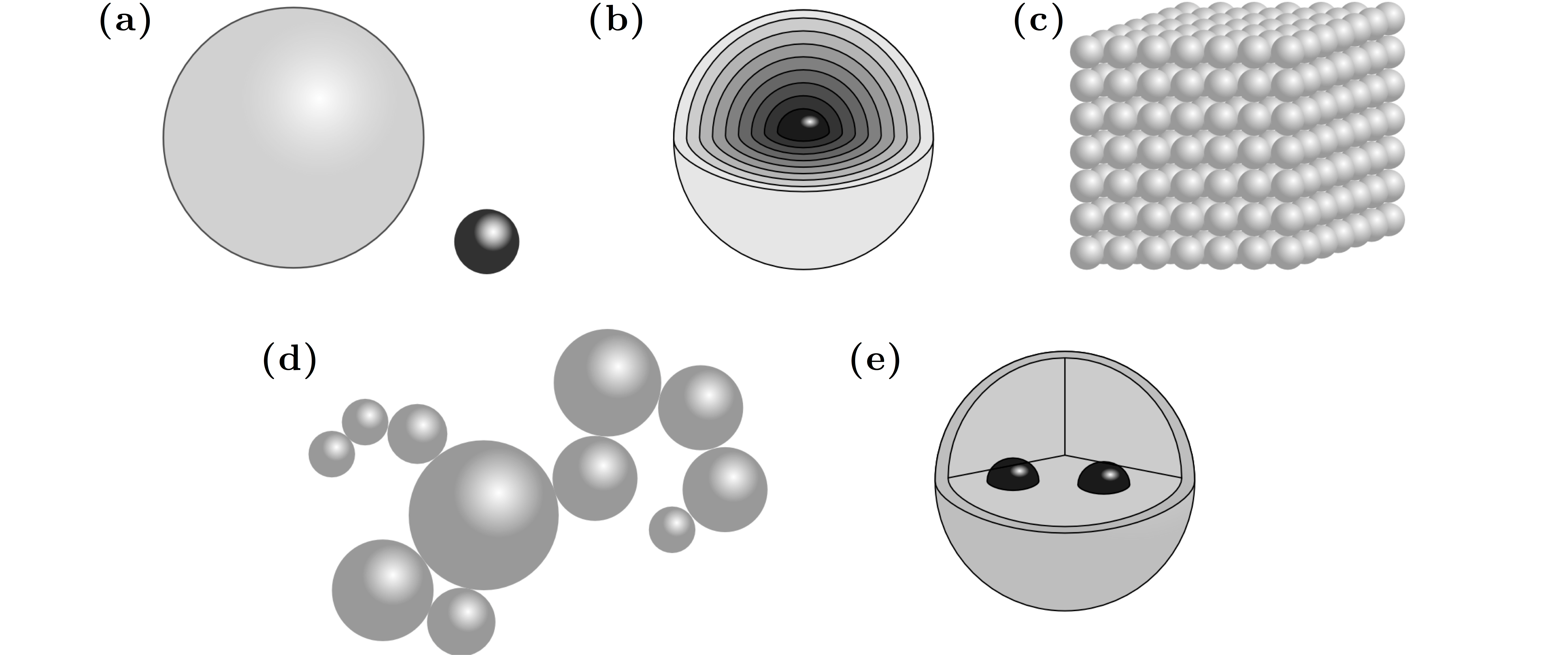# Chapter 6 — Computational Methods

As we have seen in the previous Chapters, the computation of optical forces and torques requires the solution of the optical scattering problem in order to evaluate the rate of change of linear and angular optical momenta. There is no one single right approach for all situations, rather the most appropriate computational technique depends on the system under study. For particles that are large compared with the optical wavelength, the geometrical optics approach described in Chapter 2 may be the most appropriate, while for particles much smaller than the wavelength, the dipole approximation dealt with in Chapter 3 may be a computationally efficient option. At intermediate length scales, though, rigorous solutions of Maxwell’s equations are required, as we have seen in Chapter 5. The special and extremely important case of a homogeneous spherical particle can be solved analytically thanks to Mie theory, as we have seen again in Chapter 5. Nevertheless, non-homogeneous and non-spherical particles, such as the ones shown in Fig. 6.1, are of crucial impor- tance for optical manipulation applications. This Chapter is dedicated to exploring computational techniques adequate to deal with these particles and, in particular, the T-matrix method. Other approaches will also be introduced — in particular, the discrete dipole approximation (DDA) method and the finite-difference time-domain (FDTD) method, which have the advantage of being able to model particles of ar- bitrary shape and material, but are typically regarded as extremely demanding in terms of computing resources.

6.1  T-matrix
6.1.1  Optical force
6.1.2  Optical torque
6.1.3  Amplitudes of a focused beam
6.1.4  Translation theorem
6.1.5  Rotation theorem
6.1.6  Clebsch–Gordan coefficients

6.2  Metal spheres sustaining longitudinal fields

6.4  Clusters of spheres
6.4.1  Aggregates of spheres
6.4.2  Inclusions
6.4.3  Convergence

6.5  Discrete dipole approximation

6.6  Finite-difference time domain

6.7  Hybrid techniques

Problems

References